# Binary Space Partition Algorithms

One of the classic approaches to compute indoors rendering at interactive frame rates involves using a BSP data structure. Variants of this approach have been used in the Doom and Quake series as well as in dozens of other games. Doom used a 2D BSP, whereas newer versions have moved to 3D data structures and added a host of optimizations and improvements.

A BSP is just a binary tree data structure that allows us to classify geometric data (in our case, triangles) spatially. As you will soon see, its main advantage is viewpoint-independent triangle ordering. We can query the BSP from a given waypoint, and it will return to us the list of triangles it's storing, ordered by Z-value. With some enhancements, a BSP can also be customized to help with occlusions. Unfortunately, BSPs are traditionally considered hard to explain, so we will need some in-depth analysis to understand how to harness their power.

#### Construction

The construction of a BSP begins with an unordered set of triangles, which we want to classify. The usual starting point is the whole geometry data set of a game level, for example. The algorithm is recursive and can be outlined as follows:

1. Begin with the complete set.

2. Select the triangle that best divides the set in half.

3. Compute the support plane of the said triangle.

4. Store the selected triangle and its support plane in the current node.

5. Divide the remaining triangles depending on which side of the selected plane each one stays.

6. If any of the two sublists is not empty, create a new node and go to step 2.

This is rather obscure, so let's try to shed some light on the whole construction process. I will use a 2D drawing to illustrate, so instead of 3D triangles (which are quite hard to picture on a flat page), you will see 2D lines. But the process is the same. Think of this drawing (see Figure 13.1) as a top-down view of a Quake level; each line depicting one wall.

##### Figure 13.1. Game level, top-down view.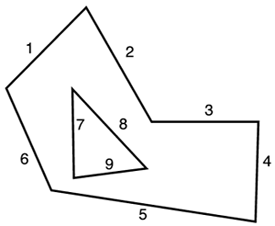The process would begin by splitting the set in half using 8 as a base (see Figure 13.2).

##### Figure 13.2. Figure 13.1 is split using 8 as a base. The front contains 1.2, 2, 3, 4, 5.2, whereas the back contains 1.1, 6, 7, 9, 5.1.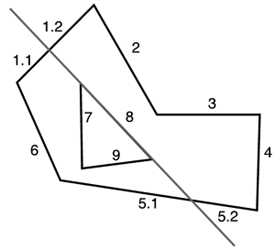Then it would split the front using 3 (see Figure 13.3).

##### Figure 13.3. After this second split, the front contains 4 and 5.2, and the back contains 2 and 1.2.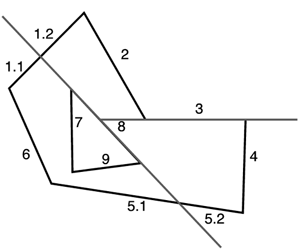The two remaining nodes can be split trivially: Given a node with two primitives (4 and 5.2, for example), one will always be the root, and the other will be one of the two children. In this case, we choose 4 as a root, and thus 5.2 is in the front node. In the second case, 1.2 is the root, and 2 is in front of it.

Thus we have the first subtree. The map is shown in Figure 13.4.

##### Figure 13.4. State of the map after the front subtree has been completely processed.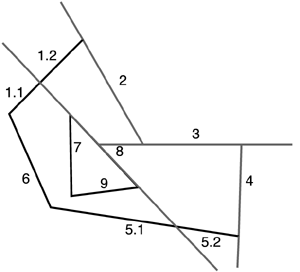The tree is shown in Figure 13.5.

##### Figure 13.5. BSP tree with the front subtree processed.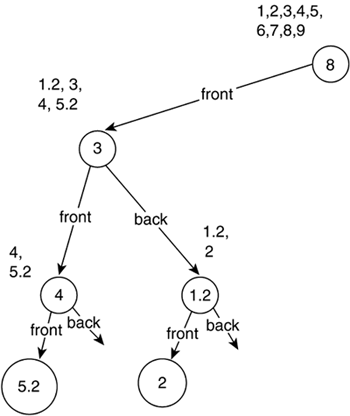Working similarly with the front node from the root, we get the tree shown in Figure 13.6.

##### Figure 13.6. Full BSP tree after completely scanning the map.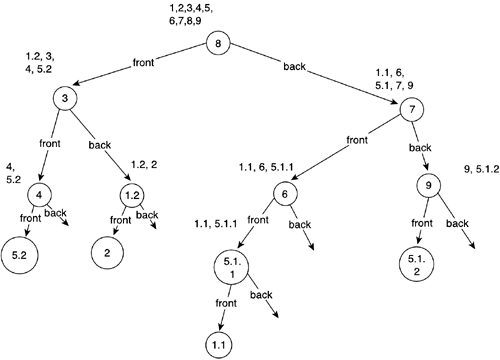Figure 13.7 illustrates what the map looks like now.

##### Figure 13.7. Resulting map after BSP construction, showcasing the partition planes.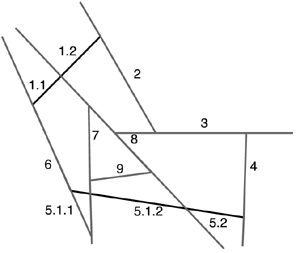BSPs just take geometrical sets and divide them recursively using support planes. In the end, each node of the BSP holds exactly one triangle, which was used to split the data set at that point. There are just two questions we need to solve in order to code the algorithm. First, we need to define the criteria of selection for the splitting plane. Second, we need to decide what to do when a triangle is divided into two halves by the splitting plane.

Choosing the ideal splitting plane can be achieved in a variety of ways. One option is to divide the set using the triangle whose support plane is closest to the center of the set, trying to make sure both subtrees have the same amount of geometry, which results in a balanced BSP tree. But such criteria can often generate lots of vertex splits, and thus the geometric data set will quickly grow, increasing our memory footprint. An alternative method consists of selecting, from the triangles located approximately in the middle (close to the center), the triangle whose support plane generates less vertex splits.

NOTE

Let's clarify what "center" means here. One option would be to use the mathematical barycenter, expressed as the average value of all points comprising the set. Thus, for a set V, the barycenter would be as follows:

` Barycenter=(S(V[i]))/i `

Although mathematically correct, this might not suit our needs completely. Because this is a subtle concept, I will provide an example. Imagine an object with vertices distributed unevenly. Think, for example, of a lollypop: Its barycenter will be displaced toward the center of the candy ball, just because that area has more vertices. Although this is correct (both sets resulting from the plane split will be equally sized in terms of number of primitives), we might run into trouble because cells created by the BSP will not be of equal size. In some games, AI developers will prefer to sacrifice tree balance in order to keep equal-sized cells. If this is your situation (your AI programmer will tell you so), a better choice for a center will be the midpoint of the bounding box of the incoming data set. This way you ensure that the set is divided in half not by number of primitives, but by its spatial allocation.

Whichever division plane you choose, the main problem with the implementation of a BSP is deciding what to do if, when splitting the geometry set, one triangle effectively crosses the support plane. The usual strategy is to split the triangle, which in general generates three new triangles one on one side of the support plane and two on the opposite side. Triangle splitting makes coding BSPs a bit more complex. The code is not that hard, but it's pretty unstable, and thus many special cases must be covered carefully. The following listing, one of the longest in the book, is the complete triangle split routine, commented. I think it is of great value because it's a pretty involved routine that hasn't been published in many books. The main idea of this implementation is to evaluate the three points in the triangle against the plane, compute the addition of these values, and use that to drive a switch construct. This way we narrow down the different cases quickly (there are 27 variants, many of them symmetrical) to achieve good performance.

` int triangle::split(plane pl,triangle *tris1,int &num1,triangle *tris2,int &num2) { int v1= pl.testpoint(p1);          // -1, 0 or 1 depending on which side we are in int v2= pl.testpoint(p2); int v3= pl.testpoint(p3); int val=v1+v2+v3; switch (val)    {    case 3:       // triangle completely in positive side       tris1=(*this);       num1=1;       num2=0;       return 1;    case -3:       // triangle completely in negative side       tris2=(*this);       num1=0;       num2=1;       return 1;    case -2:       // triangle with two vtx on the plane and the other on negative side       // no need to divide it       tris2=(*this);       num1=0;       num2=1;       return 1;    case 2:       // triangle with two vtx on the plane and the other on positive side       // no need to divide it       tris1=(*this);       num1=1;       num2=0;       return 1;    case 0:       // triangle in plane or triangle with one vertex in plane and       // other two in opposite sides. The latter requires a divide.       if (v1 || v2 || v3) // two vertices on opposite sides... divide          {          point pivot, positive, negative;          if (v1==0)             {             pivot=p1;             if (v2>0) { positive=p2; negative=p3; }             else { positive=p3; negative=p2; }             }          if (v2==0)             {             pivot=p2;             if (v1>0) { positive=p1; negative=p3; }             else { positive=p3; negative=p1; }             }          if (v3==0)             {             pivot=p3;             if (v1>0) { positive=p1; negative=p2; }             else { positive=p2; negative=p1; }             }          // here positive, pivot and negative are ready          point i;          pl.testline(positive,negative,i);          tris1.create(positive,i,pivot);          num1=1;          tris2.create(negative,pivot,i);          num2=1;          return 2;          }       else // triangle is inside plane... assign to positive node          {          tris1=(*this);          num1=1;          num2=0;          return 1;          }       break;    case -1:       // can be: two vtx on plane and one on negative side, one vertex       // on positive and two vertices on negative. Latter requires a divide       if (v1*v2*v3==0) // one of them was zero: we're on the first case          {          tris2=(*this);          num1=0;          num2=1;          return 1;          }       // one vertex on positive, two on negative. Split       point positive,negative1,negative2;       if (v1==1) { positive=p1; negative1=p2; negative2=p3;}       if (v2==1) { positive=p2; negative1=p1; negative2=p3;}       if (v3==1) { positive=p3; negative1=p1; negative2=p2;}       point v1=negative1-positive;       point v2=negative2-positive;       point i1,i2;       pl.testline(negative1,positive,i1);       pl.testline(negative2,positive,i2);       tris1.create(positive,i1,i2);       num1=1;       tris2.create(negative2,i2,i1);       tris2.create(negative2,i1,negative1);       num2=2;       return 3;    case 1:       // can be: two vtx on plane and one on positive side, one vertex       // on negative and two vertices on positive.Latter requires a divide       if (v1*v2*v3==0) // one of them was zero: we're on the first case          {          tris1=(*this);          num1=1;          num2=0;          return 1;          }       // one vertex on negative, two on positive. Split       point positive1,positive2,negative;       if (v1==-1) { negative=p1; positive1=p2; positive2=p3;}       if (v2==-1) { negative=p2; positive1=p1; positive2=p3;}       if (v3==-1) { negative=p3; positive1=p1; positive2=p2;}       point v1=positive1-negative;       point v2=positive2-negative;       point i1,i2;       pl.testline(positive1,negative,i1);       pl.testline(positive2,negative,i2);       tris1.create(positive1,i1,i2);       tris1.create(positive1,i2,positive2);       num1=2;       tris2.create(negative,i2,i1);       num2=1;       return 3;    } } `

It's a very lengthy routine. But if you examine it carefully, you will discover that the execution paths rarely run more than a few instructions.

Once we have the triangle splitting routine, we need the overall definition of the BSP tree:

` class bsp; class bsp    {    bsp *leftnode;    bsp *rightnode;    plane supportplane;    triangle tri;    public:       void create(list<triangle>);    }; `

Then, creating the BSP is just a routine:

` select best support plane for each triangle in the input set    split it    arrange the resulting triangles in a front and back list if front list is not empty    call create with this front list if back list is not empty    call create with this back list `

#### View-Dependent Sorting

You have now seen how to build a BSP data structure. It is a complex, counterintuitive process with no apparent goal. From the building algorithm, it is hard to imagine how useful BSP trees can really be. As a first glimpse toward its benefits, consider the following algorithm:

` begin with a viewpoint, and at the root node of the tree test if we are "in front" or "behind" the plane if we are in front:    scan the back node    paint the triangle which divides the set    scan the front node else    scan the front node    paint the triangle which divides the set    scan the back node `

If we execute this algorithm recursively on the BSP in the previous section, using the viewpoint as specified in the figure, the order in which the triangles will be painted is shown in Figure 13.8.

##### Figure 13.8. BSP example for view-dependent sorting. Triangles are painted (back to front) as follows: 6, 5.1.1, 1.1, 5.1.2, 9, 8, 1.2, 2, 3, 4, 5.2.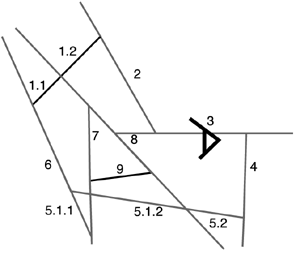Note how the list enumerates all the triangles in the set in Z-order from back to front while keeping a linear cost. The capability of ordering triangles back to front was an immediate benefit from BSPs, because it eliminated the need for a Z-buffer at a time when Z-buffers were implemented in software at a very high computational cost. If you want to understand why a BSP can order in linear time, consider this explanation: We basically scan the tree, and we keep selecting the most distant nodes from the available ones. So, each node we paint will be a bit closer to the viewpoint than the already painted ones, but not closer than any other.

Clearly, the complex construction algorithm was worth it. However, Z-ordering is less important today than in the 1980s when BSPs were introduced. We have 3D accelerators that come with built-in hardware Z-buffers, right? Well, not really. By reversing the algorithm just explained, we can achieve front-to-back order as well, and that's a blessing for a modern video card. Video cards usually perform a set of operations on each pixel:

` Test if the pixel's Z-value affects the Z-buffer If it doesn't, reject the pixel and move on to the next If it does, Compute texture mapping Compute interpolated colors Compute lighting Mix these to generate a single pixel color Paint the pixel Update the Z-buffer `

Quite clearly, the "accept pixel" path is much longer than the "reject pixel" path. Pixels that are rejected by the Z-buffer early in the process take less time from the GPU. Painting back-to-front in such an architecture actually hurts performance. Each new triangle will cover old triangles, so there will be lots of "pixel accept" paths. If you reverse the order, large, close triangles enter the Z-buffer first and make sure many subsequent calls fail due to early Z-rejection. As a result, most modern 3D accelerators experience a noticeable performance increase if you paint primitives front-to-back, and this is something a BSP can definitely help you with.

#### Hierarchical Clipping

BSPs can easily be extended to accelerate clipping computations, which allow us to eliminate the geometry that effectively lies outside of the view frustum. The key concept here is really simple: As part of the construction process, compute a bounding volume for each tree node. Clearly, successive levels in the BSP will have smaller bounding volumes because the partition condition of the tree guarantees that each node will consist of a spatially tighter triangle set. Besides, if the planes effectively divide sets in half, we can ensure that this bounding box hierarchy will divide box sizes in half approximately, with each new iteration.

Now it is just a matter of using these bounding boxes as part of the traversal algorithm, which is explained in the next section. The required modification works as follows:

` begin with a viewpoint, and at the root node of the tree if the current node's bounding box is visible test if we are "in front" or "behind" the plane if we are in front:    scan the back    paint the triangle which divides the set    scan the front node else    scan the front node    paint the triangle which divides the set    scan the back node end if end if `

Notice how this algorithm can prune large sections of the BSP tree very early in the rendering pipeline. As we traverse the tree, the moment one bounding box lies outside the frustum, we can reject not only that node but the complete subtree that is derived from it. Remember, boxes will be stacked hierarchically, so if an Nth level box is outside the frustum, any further box, which will be inside the initial box, will be outside as well. So, you can expect this improvement to greatly effect the overall performance of an indoors renderer. Remember, we are talking about game levels where the geometry surrounds the player, so there are lots of opportunities for clipping geometry that's behind him. Additionally, you can enhance the "is visible" test with a Z-rejection step. If you know the upper bound of the visibility range (distance at which geometry can be seen), any bounding box located further than that can definitely be pruned along with its complete subtree. If you review the code, you will see that our clipping processor has a huge impact, consuming very little CPU time in the process just a few box-in-frustum tests.

#### Occlusion Detection

BSPs can also be used to compute occlusion detection. But for this technique to work, it requires some additional preprocessing because a regular BSP is not well suited for these tests. A new type of BSP, called the leafy-BSP, needs to be introduced at this point. Leafy-BSPs are at the core of Quake's rendering pipeline, which is the program that made them quite popular for game developers. They have the property of automatically dividing the incoming data set in an array of convex cells, which will prove very useful.

A leafy-BSP is a regular BSP where all the geometry has been propagated to the leaves. In a regular BSP, data is stored in internal nodes in the form of triangles that divide the triangle sets. So, leaf nodes really only store the last triangle that survived the plane division approach. Now we will need to transform this BSP to leafy form by "shaking" the tree from the root so all triangles in internal nodes are propagated to the leaves. You can see the leafy process applied to our classic BSP tree in Figure 13.9.

##### Figure 13.9. Leafy-BSP, showing the contents of each leaf node.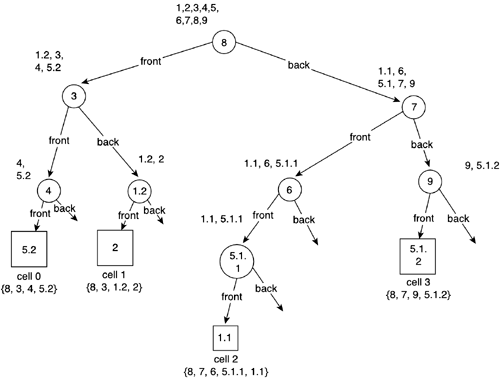Once we have propagated the data, we can forget about the tree for a second and store the triangle list of each node in a list of numbered cells. The list for our previous example, along with its geometrical representation in the game level, is shown in Figure 13.10.

##### Figure 13.10. Leafy-BSP with cells.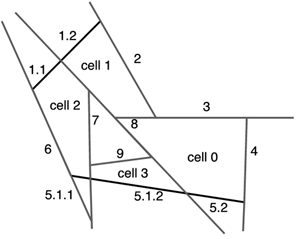Notice how each cell represents a contiguous spatial zone in our initial triangle set. Moreover, we can demonstrate that each of those cells is really a convex zone of the map, which will be very handy. The walls of the cells can either be areas occupied by level geometry or openings between cells. We will detect these openings at this point and store them along with the cells they belong to. Each opening should belong to exactly two cells.

If you analyze the process we have just gone through, you will see that we have just found an automatic algorithm to convert any triangle set into a list of convex cells and gates that connect them. The hard part is well behind us, and it is now time to take advantage of this new data structure the same way we took advantage of the classic BSP.

We will first precompute cell-to-cell visibility. Starting with one cell, we need to detect the set of cells that can be seen standing from the first one. To do so, we will use the gates (usually called portals) associated with that cell. We send rays from points in the portal toward the other portals in the level, and test whether any of them effectively reach their destination. If a single ray travels from portal A to portal B, it means the rooms connected to that portal are mutually visible. Note how visibility is a symmetrical property. This algorithm was introduced by Seth Teller and is called portal stabbing. As you will learn in the next section, there is a slightly different approach called portal rendering, which somehow has the same starting point.

Additionally, we will store the visibility information in a data structure called a Potentially Visible Set (PVS). A PVS is just a matrix of bits. If position X,Y has a value of true, then room X sees room Y. Thus, the PVS stores a summary of all the visibility processing. This is the real meat we will be using in the rendering loop.

#### Rendering

Now that we have analyzed how to perform clipping, view-independent sorting, culling, and occlusion determination using a BSP, let's examine a complete rendering algorithm that combines all these features in a general solution. The algorithm that follows is not very different from the one used in Quake III: Arena.

We start by using the BSP to quickly determine the cell the player is standing in. We traverse the BSP and use the splitting plane to narrow down and finally reach the cell. Then, we use the PVS to determine which cells are visible from the one we are standing in. All we need to do is scan the bit masks in the PVS. Notice how, at this stage, we have already computed occlusions, which was the hardest of all computations before leafy-BSPs were introduced. Then, we need to render all the potentially visible cells. We paint front-to-back (which helps the Z-buffer reject unseen fragments as soon as possible and is thus more efficient than back-to-front), sort the cells by distance, use their bounding box to clip them to the screen, and send them to the graphics hardware.

Overall, we have taken occlusions, clipping, and culling (which will be performed on the hardware) into consideration, with almost zero visibility computations in the real-time loop. All we need to compute is the room the player is standing in and the view frustum culling of the visible cells. Besides, we know which cell the player is staying at, so we can use that information for AI, collision detection, and so on. The combination of clipping, culling, and a leafy-BSP approach for occlusion determination provides a complete rendering framework that tames the visibility problem's complexity.Core Techniques and Algorithms in Game Programming2003
ISBN: N/A
EAN: N/A
Year: 2004
Pages: 261